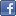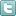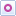EE Times-India > FPGAs/PLDs

FPGAs/PLDs# Developing application in SDSoC (Part 2)

Posted: 24 Dec 2015Print Version

Keywords:high-level synthesis  HLS  FPGA  EDA  FIR

High-Level Synthesis (HLS) has broken into the main stream with FPGA vendors and EDA companies offering tools which convert C, C++, System C and Matlab into FPGA bit streams. For hybrid SoCs, the same high-level language lets us design both the processor and the programmable logic and move design elements between the processor and FPGA fabric. Part 1 of this series looked at one such HLS tool: SDSoC from Xilinx. In that article, we asked readers to select the algorithm for implementation with SDSoC.

In answer to the question if the first SDSoC example should focus upon a filter or transfer function implementation, it seems that most readers would prefer to see the FIR filter implementation. So are going to look at how we can create that system and then how we can boost its performance in the programmable logic side of the Zynq with the click of a few buttons.

Before we can implement the algorithm we must first know what we are going to implement and design it appropriately. FIR Filters are a commonly used type of digital filter, one of the great advantages about using digital filters is that we can implement most types of responses – try doing that in analog. Of course in the digital domain we do not also suffer from the aging, tolerances, drift and temperature effect which analog components do, although there are other challenges.

We are going to implement a Finite Impulse Response (FIR) filter which compensates for a digital-to-analog convertors (DAC) sinc roll off. The roll off occurs as most DACs will hold an analog output level until the next sampling point. If you were to look at the output of a DAC you would see the output waveform is made up from a number pulses.When a DAC behaves in this manner it is called a zero order hold DAC, looking at the output within the frequency domain we would see the spectrum of the output pulse train multiplied by the Fourier transform of a rectangular pulse. Of course the Fourier transform of a rectangular pulse should be familiar to all engineer as the Sinc function which is the core of the Finite Impulse Response Filter.

What this means is the output of the DAC has an amplitude roll off as the frequency approaches the sampling frequency. The mathematics behind this arePlotting the above equation clearly demonstrates the roll off.To create a compensation filter we need to create the inverse function using the following steps
1. Calculate the Sinc response for the DAC in the frequency domain
2. Calculate the inverse response of the Sinc response3. Perform an Inverse Fast Fourier Transform of the inverse response
4. Plot the impulse response from the IFFT5. Obtain the filter coefficients symmetrically around the centre point of the IFFT from the impulse responseRelated Articles Editor's Choice
Comment on "Developing application in SDSoC (Par..."

Top Ranked Articles

WebinarsVisit Asia Webinars to learn about the latest in technology and get practical design tips.

Search EE Times India
Services

Go to topConnect on FacebookFollow us on TwitterFollow us on Orkut
﻿